Search by Topic

Resources tagged with Differentiation similar to Bridge Builder:

Filter by: Content type:
Age range:
Challenge level:

There are 16 results

Broad Topics > Calculus > DifferentiationImpedance Can Be Complex!

Age 16 to 18 Challenge Level:

Put your complex numbers and calculus to the test with this impedance calculation.Ramping it Up

Age 16 to 18 Challenge Level:

Look at the calculus behind the simple act of a car going over a step.Bend

Age 16 to 18 Challenge Level:

What is the longest stick that can be carried horizontally along a narrow corridor and around a right-angled bend?Integration Matcher

Age 16 to 18 Challenge Level:

Can you match the charts of these functions to the charts of their integrals?Operating Machines

Age 16 to 18 Challenge Level:

What functions can you make using the function machines RECIPROCAL and PRODUCT and the operator machines DIFF and INT?Bird-brained

Age 16 to 18 Challenge Level:

How many eggs should a bird lay to maximise the number of chicks that will hatch? An introduction to optimisation.Loch Ness

Age 16 to 18 Challenge Level:

Draw graphs of the sine and modulus functions and explain the humps.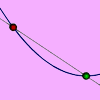An Introduction to Differentiation

Age 14 to 18

An article introducing the ideas of differentiation.Slide

Age 16 to 18 Challenge Level:

This function involves absolute values. To find the slope on the slide use different equations to define the function in different parts of its domain.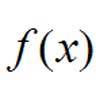Towards Maclaurin

Age 16 to 18 Challenge Level:

Build series for the sine and cosine functions by adding one term at a time, alternately making the approximation too big then too small but getting ever closer.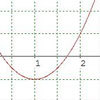Turning to Calculus

Age 16 to 18 Challenge Level:

Get started with calculus by exploring the connections between the sign of a curve and the sign of its gradient.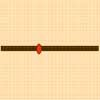Least of All

Age 16 to 18 Challenge Level:

A point moves on a line segment. A function depends on the position of the point. Where do you expect the point to be for a minimum of this function to occur.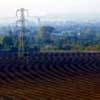Quick Route

Age 16 to 18 Challenge Level:

What is the quickest route across a ploughed field when your speed around the edge is greater?Calculus Countdown

Age 16 to 18 Challenge Level:

Can you hit the target functions using a set of input functions and a little calculus and algebra?Generally Geometric

Age 16 to 18 Challenge Level:

Generalise the sum of a GP by using derivatives to make the coefficients into powers of the natural numbers.Exponential Trend

Age 16 to 18 Challenge Level:

Find all the turning points of y=x^{1/x} for x>0 and decide whether each is a maximum or minimum. Give a sketch of the graph.